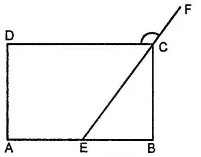Explain how this figure is a trapezium. Which of its two sides are parallelAsked by Aaryan | 1 year ago |  130

##### Solution :-

If a transversal line is intersecting two given lines such that the sum of the measures of the angles on the same side of transversal is 180º, then the given two lines will be parallel to each other.

Here, ∠ NML + ∠ MLK = 180°

Hence,NM||LK

As quadrilateral KLMN has a pair of parallel lines, therefore, it is a trapezium.

Answered by Sakshi | 1 year ago

### Related Questions

#### In the given figure, PQRS is a kite. Find the values of x and y.

In the given figure, PQRS is a kite. Find the values of x and y.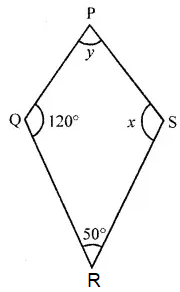#### In the given isosceles trapezium ABCD, ∠C = 102°. Find all the remaining angles of the trapezium.

In the given isosceles trapezium ABCD, ∠C = 102°. Find all the remaining angles of the trapezium.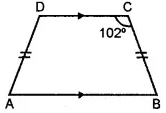#### In the given figure, ABCD is a rhombus and ∠ABD = 50°. Find :

In the given figure, ABCD is a rhombus and ∠ABD = 50°. Find :

(i) ∠CAB

(ii) ∠BCD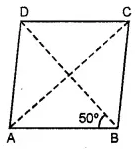#### In the given figure, ABCD is a rectangle and diagonals intersect at O. If ∠AOB = 118°, find

In the given figure, ABCD is a rectangle and diagonals intersect at O. If ∠AOB = 118°, find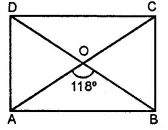(i) ∠ABO

(iii) ∠OCB